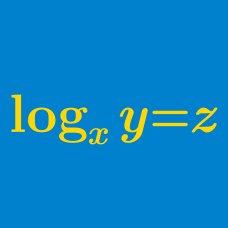Algebra

# Logarithmic Functions: Level 1 Challenges

$\large \log_7 35 + \log_7 35 - \log_7 25 = \ ?$

$\large\log_{\color{#20A900}{y}}{\color{#3D99F6}{x}}+\log_{\color{#3D99F6}{x}}{\color{#20A900}{y}}=-2$ Find the value of $\color{#3D99F6}{x}\color{#20A900}{y}.$

If $4 \log_{12} \left(x^9\right) + \log_{12} \left(x^6\right) = 126$, find $x.$

$\frac{1}{\log_{2}{100!}}+\frac{1}{\log_{3}{100!}}+\frac{1}{\log_{4}{100!}}+\ldots+\frac{1}{\log_{100}{100!}}= \ ?$

Find the smallest number among the following numbers:

(A) $\log_{2015}2016$

(B) $\log_{2016}2017$

(C) $\log_{2017}2018$

(D) $\log_{2018}2019$

(E) $\log_{2019}2020$

×